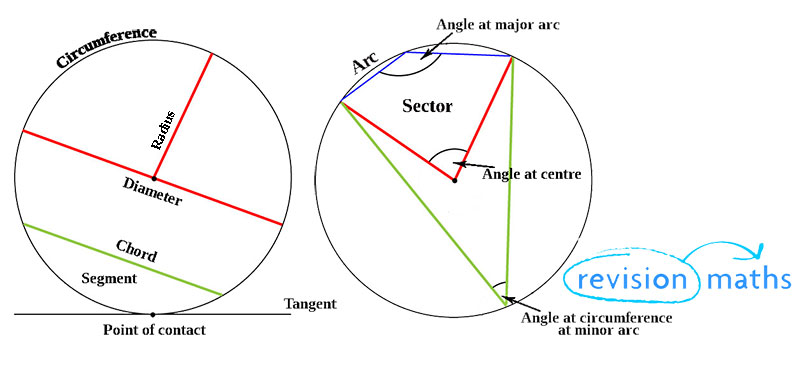## Circle Definitions

This section of Revision Maths defines many terms in relation to circles, including: Circumference, Diameter, Radius, Chord, Segment, Tangent, Point of contact, Arc, Angles on major and minor arcs, Angle of Centre and Sectors.

Circumference: The circumference of a circle is the distance around it.

Diameter: Any straight line that passes through the centre of the circle to two points on the perimeter.

Radius: Any straight line that originates at the centre of a circle and ends at the perimeter.

Chord: A straight line whose ends are on the perimeter of a circle. A diameter is the longest chord possible.

Segment: A part of the circle separated from the rest of a circle by a chord.

Tangent: A tangent to a circle is a straight line which touches the circle at only one point (so it does not cross the circle - it just touches it).

Point of contact: Where a tangent touches a circle.

Arc: A part of the curve along the perimeter of a circle.

Angle on major arc: The larger of 2 angles when a circle is split into 2 uneven parts. Greater than 180 degrees.

Angle of centre: An angle at the centre of a triangle between two lines that intersect with the perimeter.

Angle at circumference on minor arc: The smaller of 2 angles when a circle is split into 2 uneven parts. Less than 180 degrees.

Sector: A portion of a circle resembling a 'slice of pizza'.More information on Circles can be found on the Circle Theorems page Here.